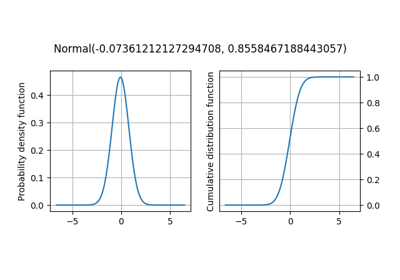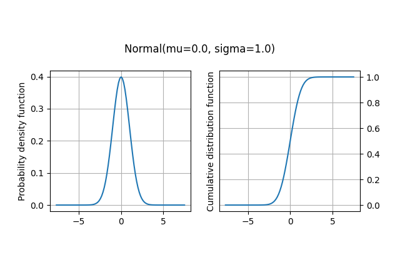gemseo / uncertainty / distributions / openturns

# distribution module¶

Class to create a probability distribution from the OpenTURNS library.

The OTDistribution class is a concrete class inheriting from Distribution which is an abstract one. OT stands for OpenTURNS which is the library it relies on.

The OTDistribution of a given uncertain variable is built from mandatory arguments:

• a variable name,

• a probability distribution name recognized by OpenTURNS,

• a set of parameters provided as a tuple of positional arguments filled in the order specified by the OpenTURNS constructor of this probability distribution.

Warning

The probability distribution parameters must be provided according to the signature of the openTURNS classes. Access the openTURNS documentation.

The constructor has also optional arguments:

• a variable dimension (default: 1),

• a standard representation of these parameters (default: use the parameters provided in the tuple),

• a transformation of the variable (default: no transformation),

• lower and upper bounds for truncation (default: no truncation),

• a threshold for the OpenTURNS truncation tool (more details).

class gemseo.uncertainty.distributions.openturns.distribution.OTDistribution(variable, interfaced_distribution, parameters, dimension=1, standard_parameters=None, transformation=None, lower_bound=None, upper_bound=None, threshold=0.5)[source]

Bases: Distribution

OpenTURNS probability distribution.

Create a probability distribution for an uncertain variable from its dimension and probability distribution name and properties.

Example

>>> from gemseo.uncertainty.distributions.openturns.distribution import (
...     OTDistribution
... )
>>> distribution = OTDistribution('x', 'Exponential', (3, 2))
>>> print(distribution)
Exponential(3, 2)

Parameters:
• variable (str) – The name of the random variable.

• interfaced_distribution (str) – The name of the probability distribution, typically the name of a class wrapped from an external library, such as "Normal".

• parameters (ParametersType) – The parameters of the probability distribution.

• dimension (int) –

The dimension of the random variable.

By default it is set to 1.

• standard_parameters (StandardParametersType | None) – The standard representation of the parameters of the probability distribution.

• transformation (str | None) – A transformation applied to the random variable, e.g. $$\sin(x)$$. If None, no transformation.

• lower_bound (float | None) – A lower bound to truncate the probability distribution. If None, no lower truncation.

• upper_bound (float | None) – An upper bound to truncate the probability distribution. If None, no upper truncation.

• threshold (float) –

A threshold in [0,1].

By default it is set to 0.5.

distribution

alias of ComposedDistribution

compute_cdf(vector)[source]

Evaluate the cumulative density function (CDF).

Evaluate the CDF of the components of the random variable for a given realization of this random variable.

Parameters:

vector (Iterable[float]) – A realization of the random variable.

Returns:

The CDF values of the components of the random variable.

Return type:

ndarray

compute_inverse_cdf(vector)[source]

Evaluate the inverse of the cumulative density function (ICDF).

Parameters:

vector (Iterable[float]) – A vector of values comprised between 0 and 1 whose length is equal to the dimension of the random variable.

Returns:

The ICDF values of the components of the random variable.

Return type:

ndarray

compute_samples(n_samples=1)[source]

Sample the random variable.

Parameters:

n_samples (int) –

The number of samples.

By default it is set to 1.

Returns:

The samples of the random variable,

The number of columns is equal to the dimension of the variable and the number of lines is equal to the number of samples.

Return type:

ndarray

dimension: int

The number of dimensions of the random variable.

distribution_name: str

The name of the probability distribution.

marginals: list[ot.Distribution]

The marginal distributions of the components of the random variable.

math_lower_bound: ndarray

The mathematical lower bound of the random variable.

math_upper_bound: ndarray

The mathematical upper bound of the random variable.

property mean: ndarray

The analytical mean of the random variable.

num_lower_bound: ndarray

The numerical lower bound of the random variable.

num_upper_bound: ndarray

The numerical upper bound of the random variable.

parameters: tuple[Any] | dict[str, Any]

The parameters of the probability distribution.

property standard_deviation: ndarray

The analytical standard deviation of the random variable.

standard_parameters: dict[str, str] | None

The standard representation of the parameters of the distribution, used for its string representation.

transformation: str

The transformation applied to the random variable, e.g. ‘sin(x)’.

variable_name: str

The name of the random variable.

## Examples using OTDistribution¶Fitting a distribution from data based on OpenTURNS

Fitting a distribution from data based on OpenTURNSProbability distributions based on OpenTURNS

Probability distributions based on OpenTURNS